Rotational Symmetry

Chapter 12 Class 7 Symmetry
Concept wise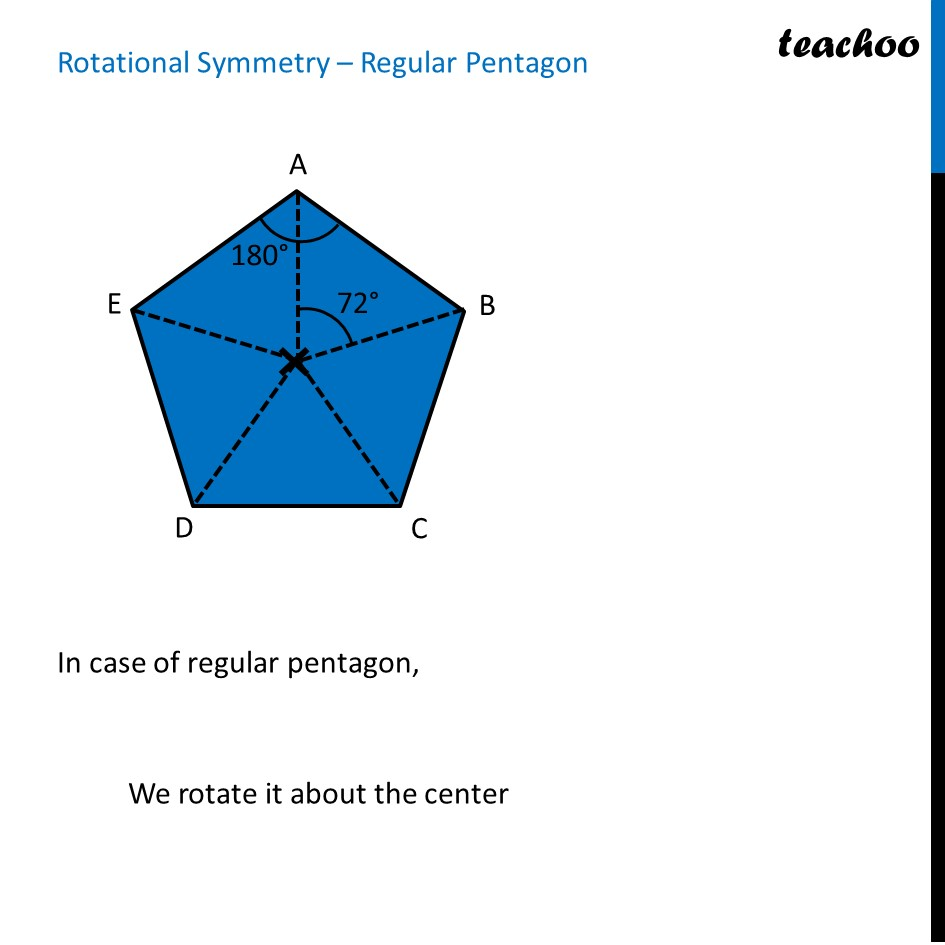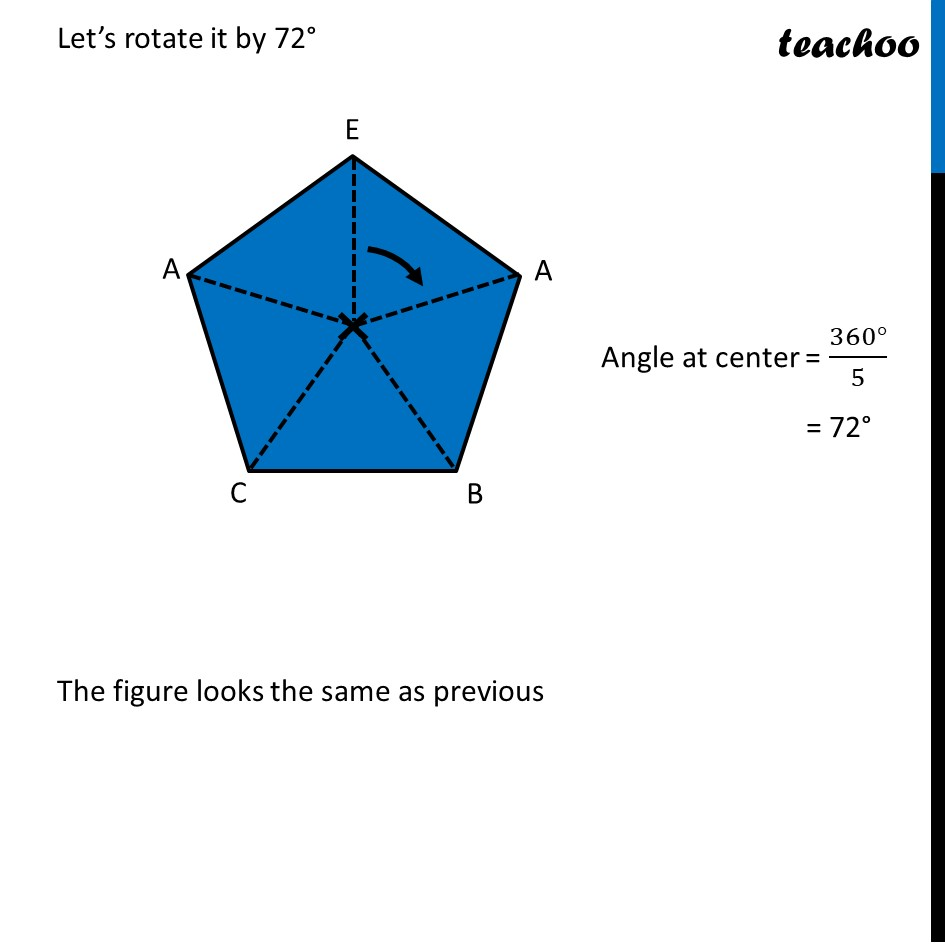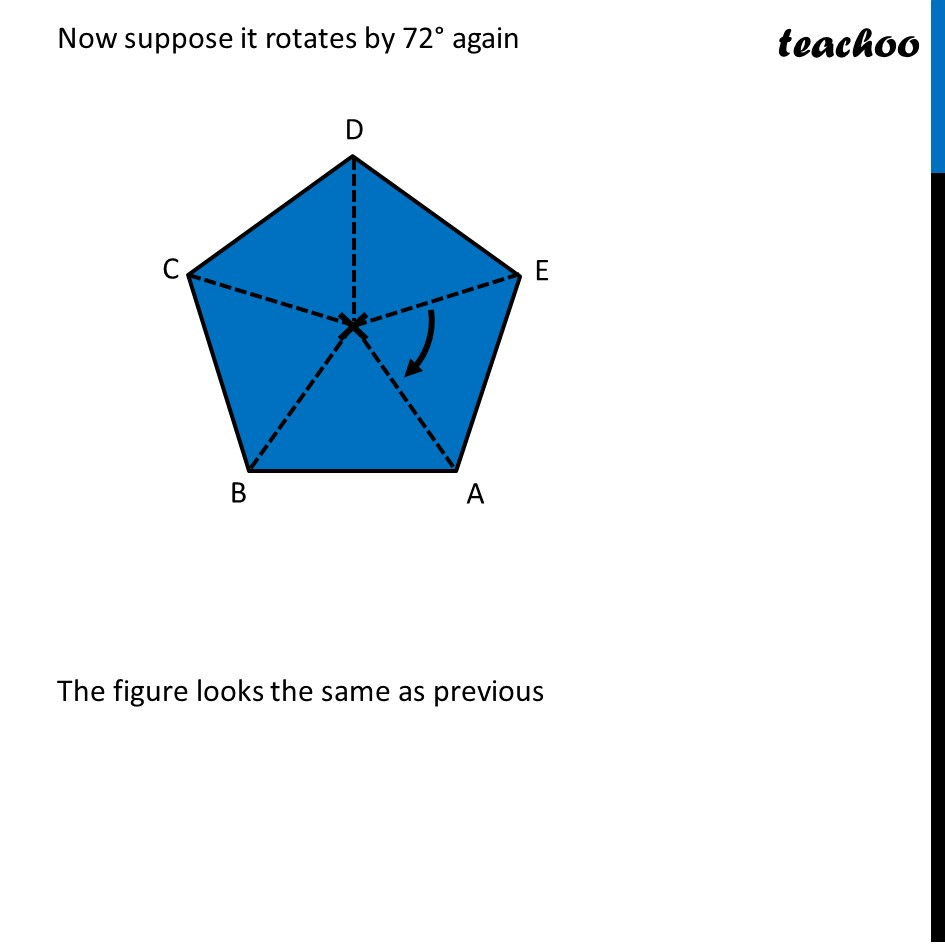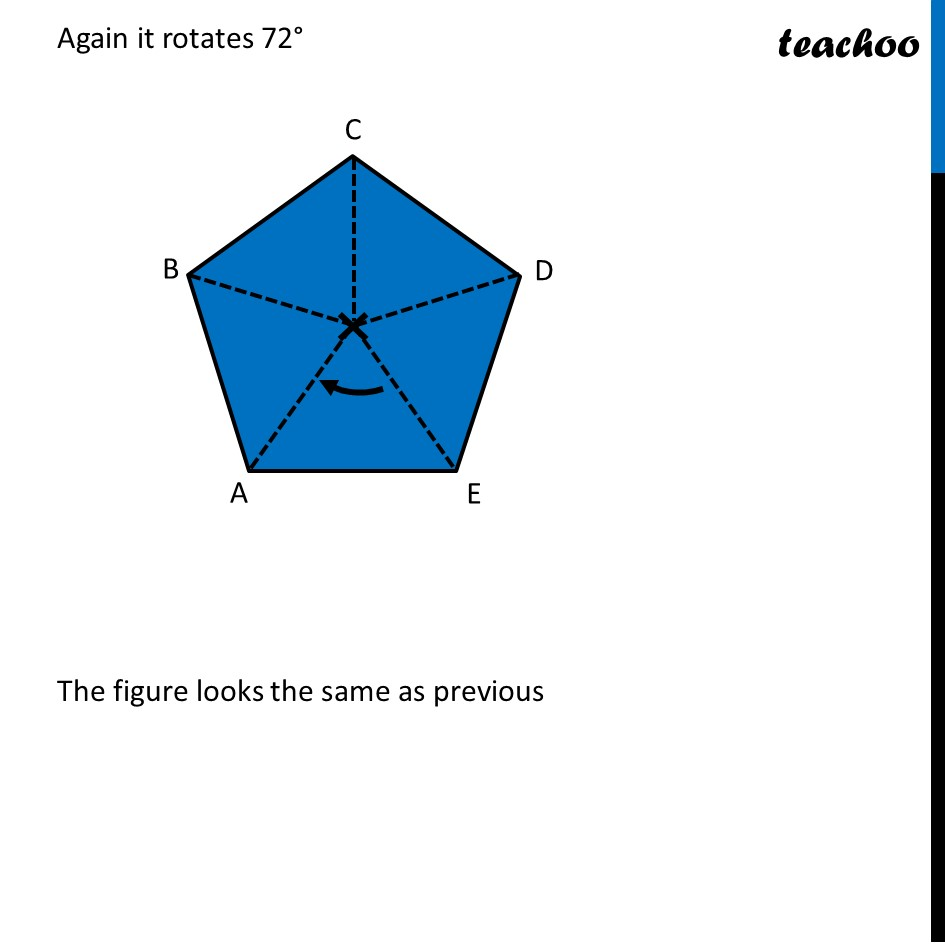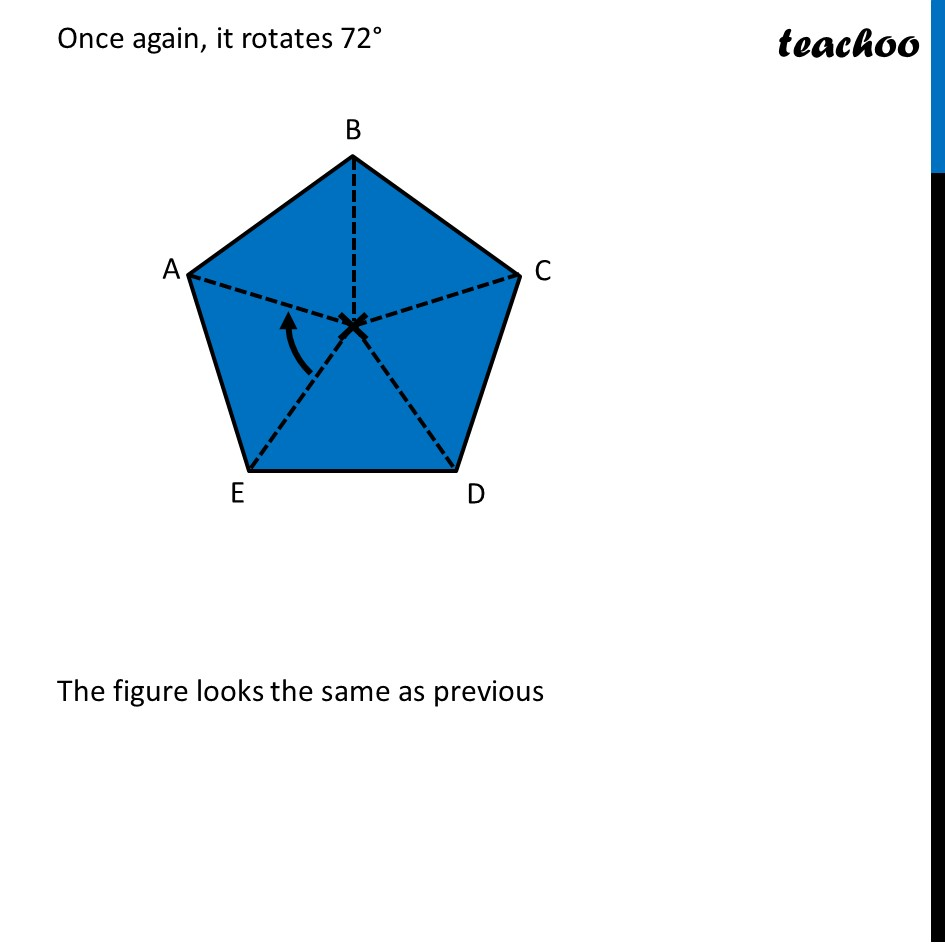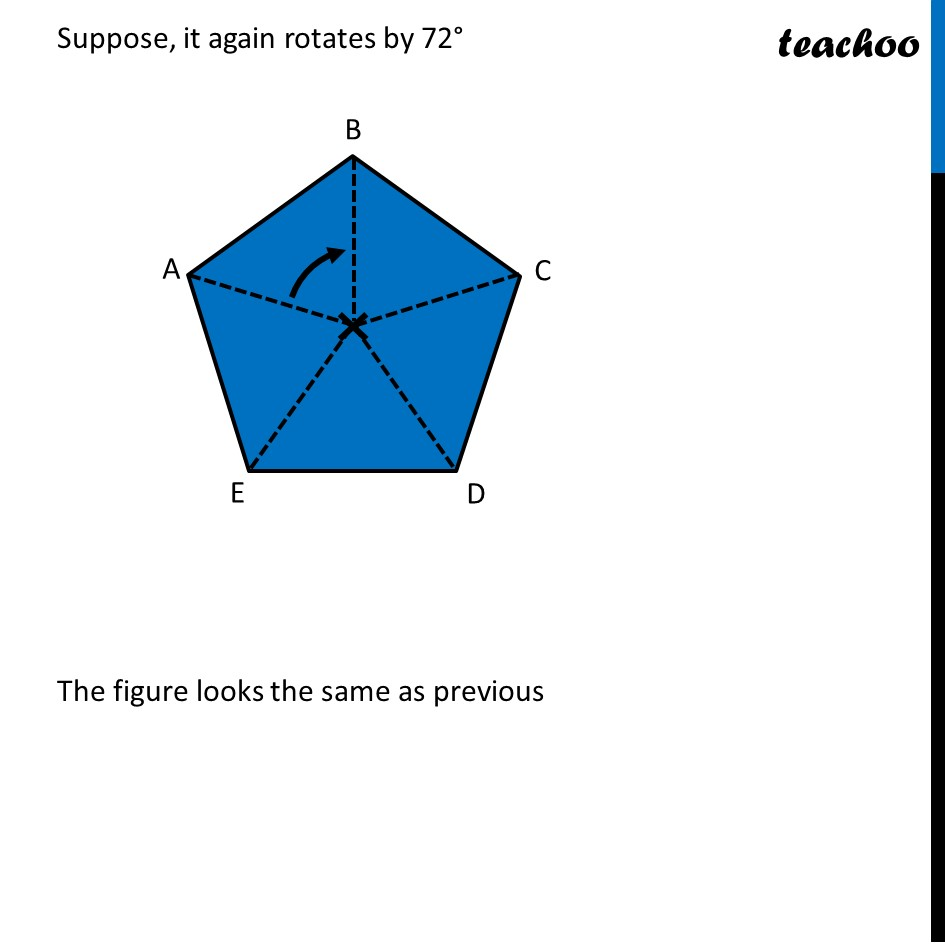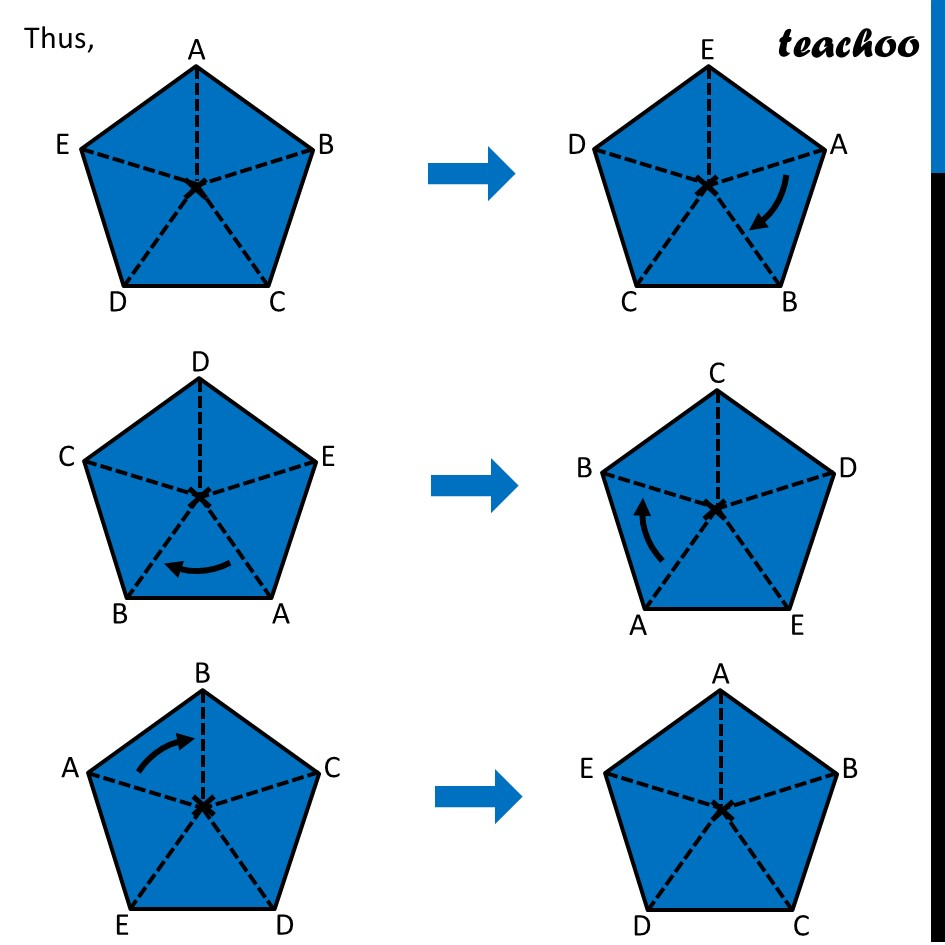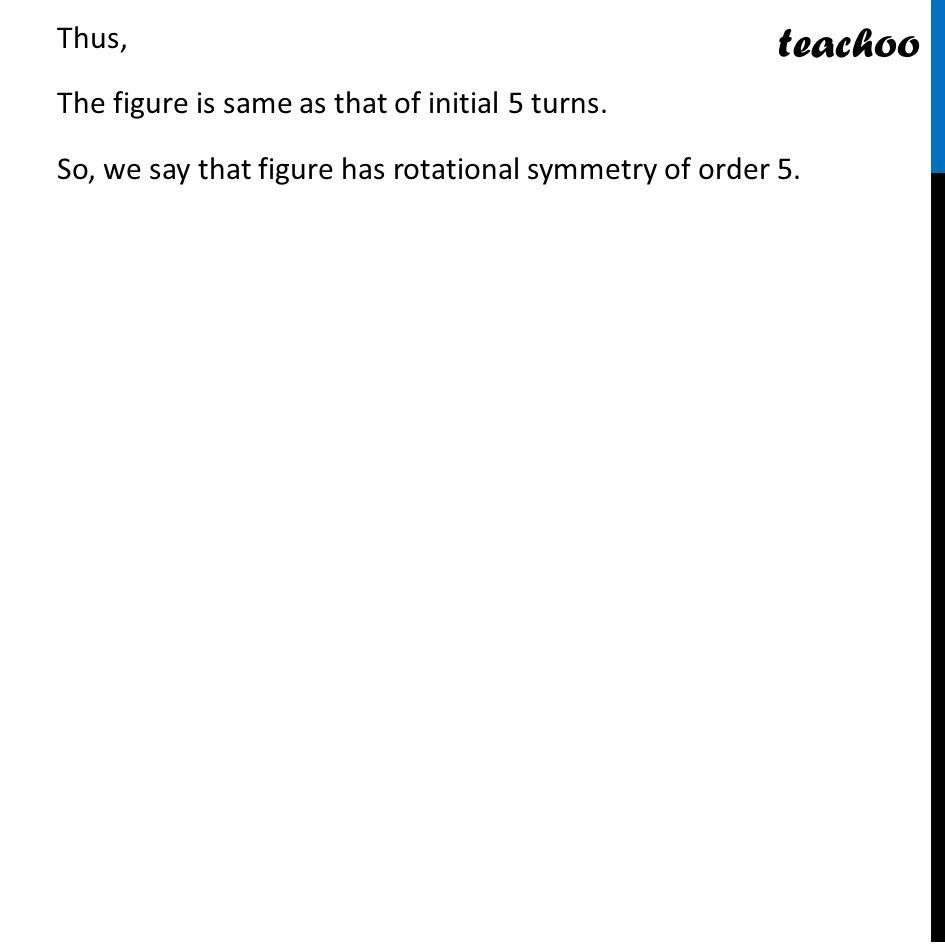Learn in your speed, with individual attention - Teachoo Maths 1-on-1 Class

### Transcript

Rotational Symmetry – Regular PentagonIn case of regular pentagon, We rotate it about the center Let’s rotate it by 72° The figure looks the same as previous Angle at center = (360°)/5 = 72° Now suppose it rotates by 72° again The figure looks the same as previous Again it rotates 72° The figure looks the same as previous Again it rotates 72° The figure looks the same as previous Once again, it rotates 72° The figure looks the same as previous Suppose, it again rotates by 72° The figure looks the same as previous Thus, Thus, The figure is same as that of initial 5 turns. So, we say that figure has rotational symmetry of order 5.